# 架构师训练营第 5 周 - 一致性 hash 算法总结及作业1、我们以图片的名称作为访问的key

2、图片的名称是不重复的，这样经过hash计算之后，都会得到一个不同的结果值

3、计算公式为：hash(图片名称)%N，N代表缓存服务器的数量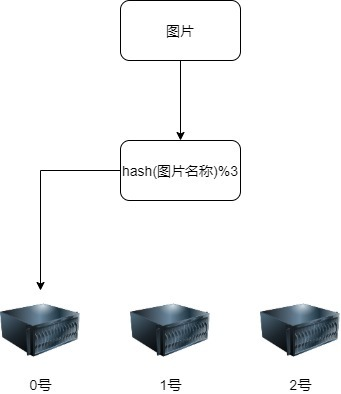1、取模的N是取的缓存服务器的数量，这样就和缓存服务器强耦合，如果增(删)缓存服务器，由于除数变化，被除数不变，就会造成余数发生变化，余数变化，则以前和缓存服务器之间的映射就失效了。放在以上的应用场景中就是：大量的图片通过hash取模后不能映射到缓存服务器（也会有一些图片会存在有效映射），那么就只有从后端去拿图片，这样在高并发的场景下，就会造成成缓存雪崩，给后端服务器造成巨大的IO压力，有可能造成整个系统瘫痪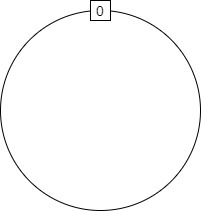1、算法公式为：hash(对象/节点)%2^32

2、将所有的缓存服务器节点结过算法计算后，会唯一对应到环上的一个点，记为:A、B、C

3、将所有需要被缓存的对象结处算法计算后，同样会对应到环上的一个点（有可能会与服务器节点合，正好就是它）,然后按顺时针查找离该对象最近的服务器节点，就是该对象缓存的宿主

1、假设现在的缓存服务器还是3台，设为：A、B、C；采用服务器Ip进行hash计算

2、需要被缓存的图片有4张，设为：P1、P2、P3、P4；采用图片名称进行hash计算

3、在经过一致性hash算法计算后，环上的分布如下图所示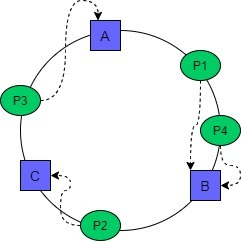1、现有缓存服务器由于访问压力大，已经快支撑不住了，因此决定再增加一台缓存服务器D

2、随机访问的图片还是原来的那4张图片，设为：P1、P2、P3、P4

3、增加缓存服务器以后，环上的分布如下图所示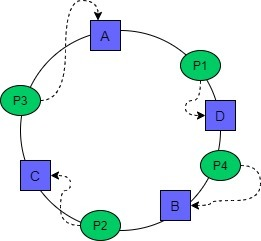1、C服务器出现硬件故障，需要更换服务器，但新服务器三天后才能到达，现在需要将C服务器从集群中下线，以免造成缓存无服务可用，需从后端拿数据

2、删除C服务器后，环上的分布情况如下：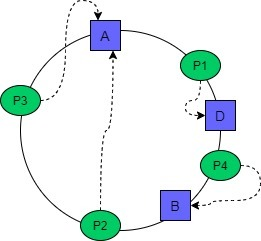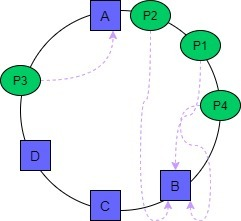what！这是什么情况，累的累死，闲的闲死。这还是一个集群吗？这不就是个人英雄主义么！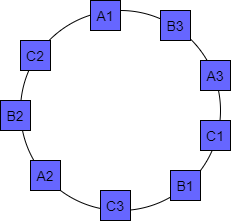1、hash算法的实现，要做到在0~2^32-1之间随机均匀分布，均匀分布得越好，数据缓存项就越均匀

2、虚拟节点的个数，理论上总共保证2^32个节点，数据分布得越均匀，但这是有代价的，所以说虚拟节点不是越多越好，要在成本和效率之间找到平衡

3、加入虚拟节点后的一致性hash计算方法调整为：hash(节点ip+虚拟节点号)%2^32

4、要保证整个一致性hash算法的效率（时间复杂度和空间复杂度）

• 用你熟悉的编程语言实现一致性 hash 算法。

• 编写测试用例测试这个算法，测试 100 万 KV 数据，10 个服务器节点的情况下，计算这些 KV 数据在服务器上分布数量的标准差，以评估算法的存储负载不均衡性。

package com.consistent.hash;import java.util.Map;import java.util.SortedMap;import java.util.TreeMap;public  abstract class DistributeHash {    private TreeMap<Long,String> virtualNodes = new TreeMap<>(); //hash=>物理节点    private int vitual_copies = 64;  //物理节点至虚拟节点的复制倍数    private Map<String,Integer> objectNodeMap = new TreeMap<>();    public abstract  Long hash(String key);    //添加物理节点    public void addPhysicalNode(String key){        for(int ind=0;ind<vitual_copies;ind++){            long hash = hash(key +"#"+Integer.toString(ind));            virtualNodes.put(hash,key);        }    }    public int getVitual_copies() {        return vitual_copies;    }    public void setVitual_copies(int vitual_copies) {        this.vitual_copies = vitual_copies;    }    public Map<String,Integer> getobjectNodeMap(){        return objectNodeMap;    }    //删除物理节点    public void removePhysicalNode(String key){        for(int ind=0 ; ind < vitual_copies ; ind++){            long hash = hash(key+"#"+Integer.toString(ind));            virtualNodes.remove(hash);        }    }    //查找对象映射的节点    private String getObjectNode(String object){        long hash = hash(object);        SortedMap<Long,String> tailmap = virtualNodes.tailMap(hash);        Long key = tailmap.isEmpty() ? virtualNodes.firstKey():tailmap.firstKey();        return virtualNodes.get(key);    }    //对象与节点映射    public void objectNodeMap(String label,int objectMin,int objectMax){        for(int object = objectMin;object <= objectMax;++object){            String nodeIp = getObjectNode(Integer.toString(object));            Integer count = objectNodeMap.get(nodeIp);            objectNodeMap.put(nodeIp,(count == null ? 0:count + 1));        }        //打印        double totalcount = objectMax - objectMin + 1;        System.out.println("======== " + label + " ========");        for(Map.Entry<String, Integer> entry : objectNodeMap.entrySet()){            long percent = (int)(100 * entry.getValue() / totalcount);            System.out.println("IP=" + entry.getKey() + ": RATE=" + percent + "%");        }    }}package com.consistent.hash;public class ConsistentHash extends DistributeHash {    // 32位的 Fowler-Noll-Vo 哈希算法    // https://en.wikipedia.org/wiki/Fowler–Noll–Vo_hash_function    @Override    public Long hash(String key) {       final int p = 16777619;       Long hash = 2166136261L;       for(int ind = 0,num = key.length();ind<num;ind++){           hash = (hash^key.charAt(ind))*p;       }       hash += hash <<13;       hash ^= hash >>7;       hash += hash <<3;       hash ^= hash >>17;       hash += hash <<5;       if(hash < 0){           hash = Math.abs(hash);       }       return hash;    }}package com.consistent.hash;import org.junit.Test;import java.util.Map;import java.util.TreeMap;public class ConsistentHashTest {    //标准方差    public double getstd(Map<String,Integer> objectnodes){        double sum = 0;        int cnt = 0;        for(Map.Entry<String,Integer> entry : objectnodes.entrySet()){            sum += entry.getValue();            cnt++;        }        double average  = sum/cnt;        int total = 0;        for(Map.Entry<String,Integer> entry : objectnodes.entrySet()){            total += (entry.getValue() - average) *(entry.getValue() - average);        }        double standardDeviation = Math.sqrt(total/cnt);        return standardDeviation;    }    @Test    public void test(){        Map<String,Integer> objectNodes = new TreeMap<>();        //添加物理服务器节点        DistributeHash ch = new ConsistentHash();        ch.setVitual_copies(102400);        ch.addPhysicalNode("192.168.100.101");        ch.addPhysicalNode("192.168.100.102");        ch.addPhysicalNode("192.168.100.103");        ch.addPhysicalNode("192.168.100.104");        ch.addPhysicalNode("192.168.100.105");        ch.addPhysicalNode("192.168.100.106");        ch.addPhysicalNode("192.168.100.107");        ch.addPhysicalNode("192.168.100.108");        ch.addPhysicalNode("192.168.100.109");        ch.addPhysicalNode("192.168.100.110");        //对象与节点映射        ch.objectNodeMap("10台服务器，单台"+ch.getVitual_copies()+"个虚拟节点",1,1000000);        //计算标准方差        objectNodes = ch.getobjectNodeMap();        System.out.println("标准方差为:"+getstd(objectNodes));    }}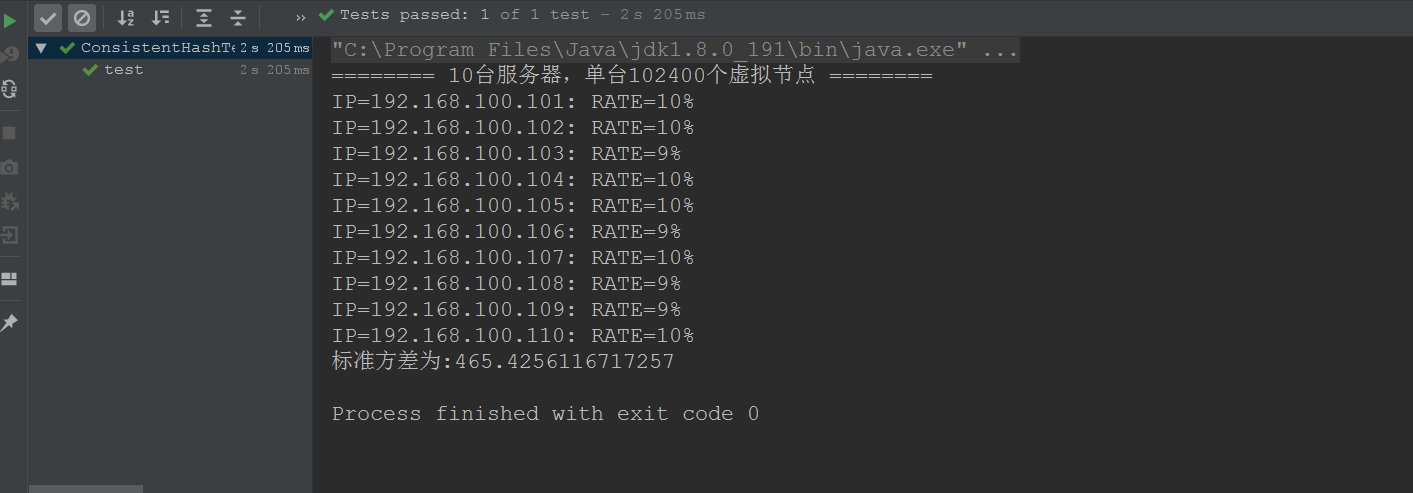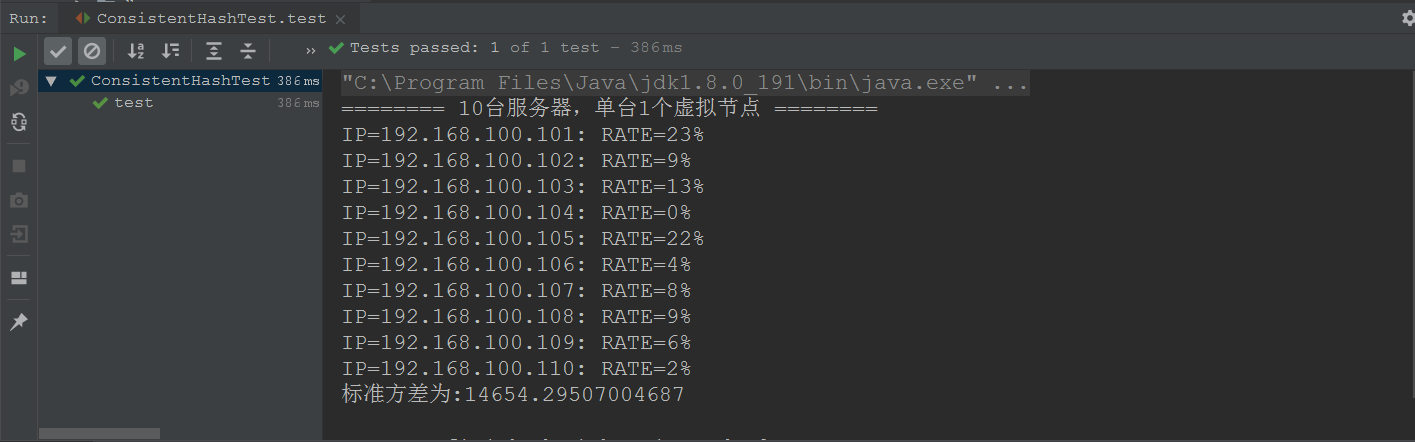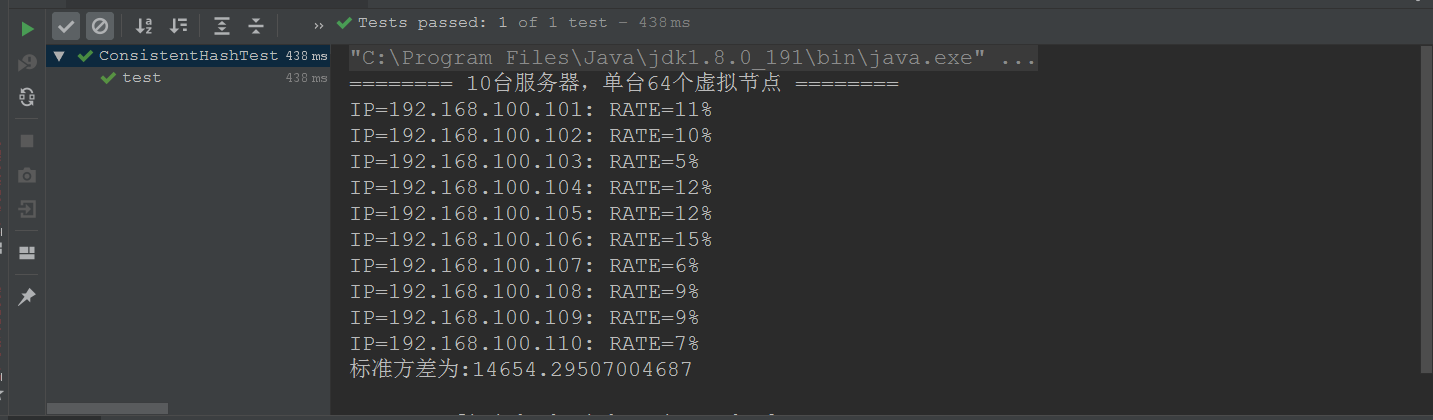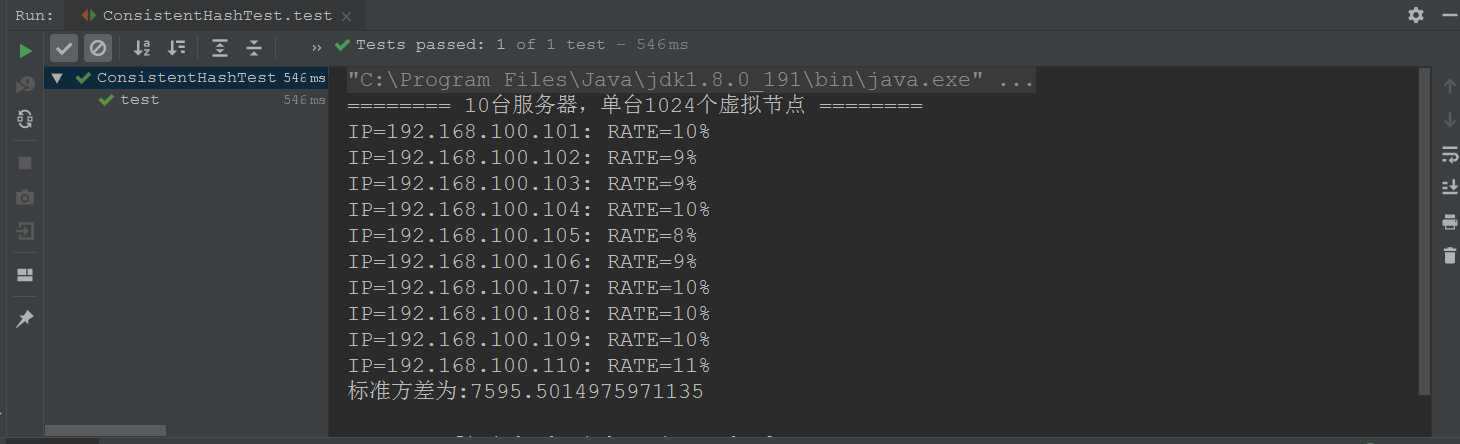## 评论